# Photofission of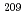Bi at intermediate energies
The reaction yields of 63 radionuclides with the mass number A = 56-135 produced in the photofission ofBi by bremsstrahlung of end-point energies (E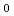) from 450 to 1100 MeV have been measured using a catcher foil technique. The charge distribution was well reproduced by a Gaussian function with the most probable charge (Z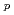) expressed by Z= RA + S, and with the A-independent FWHME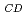. The charge distribution parameters R, S and FWHMwere independent of Eabove 600 MeV. The weighted mean values at E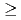600 MeV were R = 0.421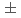0.001, S = 0.60.1 and FWHM= 2.10.1 charge unit (c.u.). Based on the charge distribution parameters, the symmetric mass yield distributions with the most probable mass Aof 961 mass unit (m.u.) and the width FWHM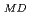of 331 m.u. were also obtained. The characteristics of the charge and mass yield distributions are discussed by referring to those for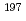Au based on the results of calculations using PICA3/GEM.